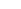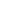# Union of Regular Grammars

## Algorithm

Given two regular grammar G1 and G2, we want to construct a regular grammar G such that L(G) = L(G1) U L(G2):

1. rename the non-terminals of G1 and G2, such that they have no common non-terminals. One possible way is to add 1 to all the non-terminals in G1, and 2 to those in G2.
2. in the regular grammar G add start symbol S and copy all rules with S1 or S2 on the left hand side to ones with S (where S1 and S2 are the start symbols in G1 and G2 respectively);
3. copy all production rules in G1 and G2 to G

## Example

Give a regular grammar recognising the union of the languages described by the following grammars:

 SaA | bB SaA | bS AaA | a | \e Aa |BbB | b |where S is the start symbol where S is the start symbol

1. Rename the non-terminals:

 S1aA1 | bB1 S2aA2 | bS2 A1aA1 | a |A2a |B1bB1 | b |where S1 is the start symbol where S2 is the start symbol

2. Create a new grammar G with start symbol S and rules starting from S and going to the right hand sides of the rules in G1 and G2 with S1 or S2 on the left hand side:

 SaA1 | bB1 | aA2 | bS2

where S is the start symbol

3. Copy all the rules in G1 and G2 to G:

 SaA1 | bB1 | aA2 | bS2 {S1}aA1 | bB1 {S2}aA2 | bS2 {A1}aA1 | a |{A2}a |{B1}bB1 | b |where S is the start symbol

## Exercises

Construct a regular grammar that recognises the union of the following pairs of languages (described using regular grammars):

1.  SaA | bC SaA | bS AaA | aB AaS | b BbB | b CcB | cC where S is the start symbol where S is the start symbol

2.  SaA | bC SaA | bS AaA | aB Aa BbB | b CcB | cC |where S is the start symbol where S is the start symbol

3.  SaA | bS SaA | bC |Aa AaA | aB BbB | b |CcB | cC where S is the start symbol where S is the start symbol

4.  SaA | bC |SAaA | aB BbB | b |CcB | cC where S is the start symbol where S is the start symbol